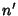## Chasles's Theorem

If two projective Pencils of curves of ordersandhave no common curve, the Locus of the intersections of corresponding curves of the two is a curve of orderthrough all the centers of either Pencil. Conversely, if a curve of ordercontains all centers of a Pencil of orderto the multiplicity demanded by Noether's Fundamental Theorem, then it is the Locus of the intersections of corresponding curves of this Pencil and one of orderprojective therewith.